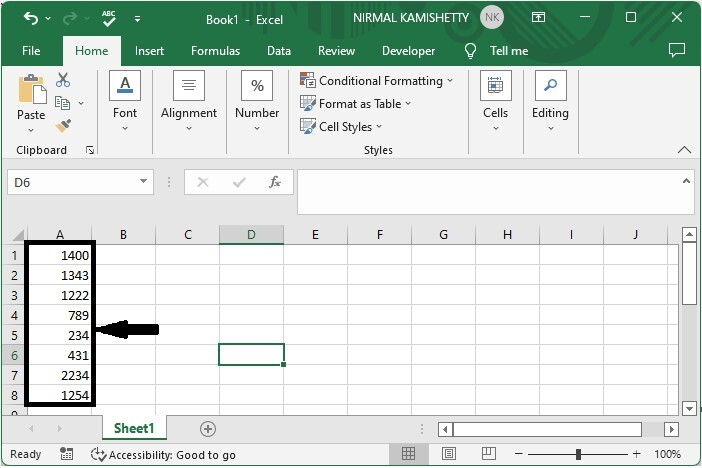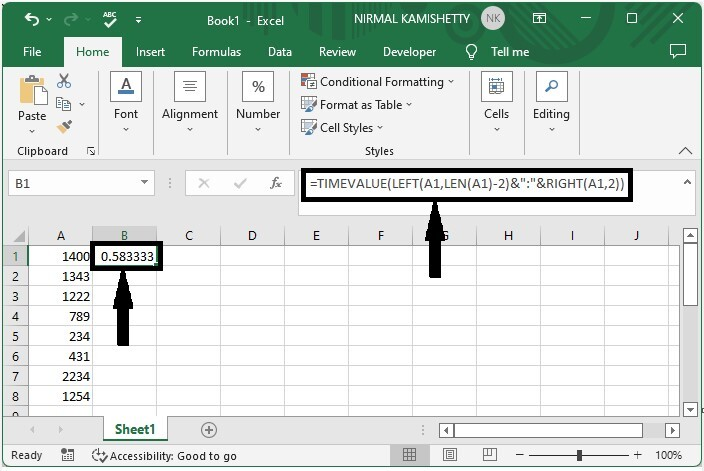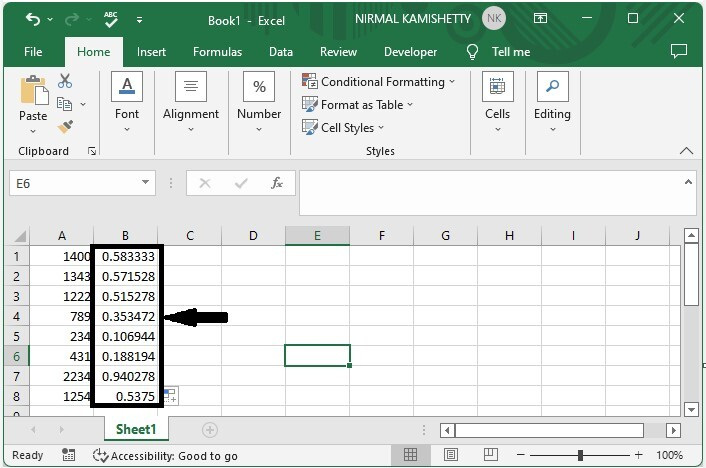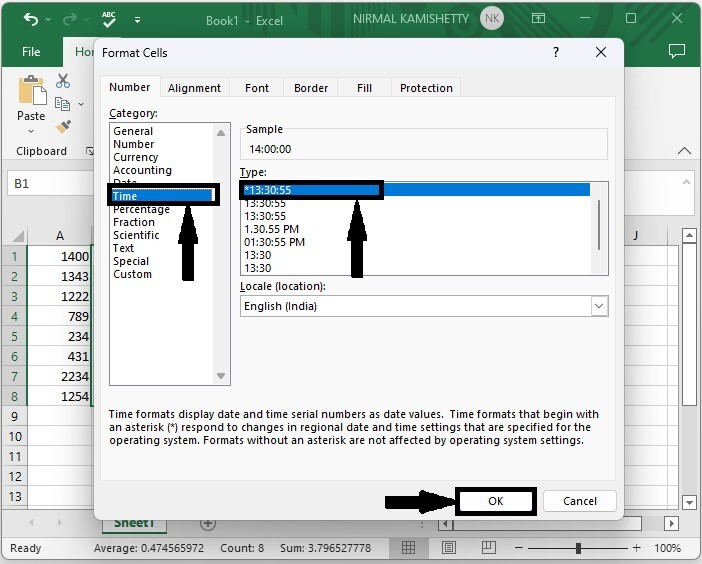# How to Convert Time String to Time in Excel?

Sometimes in Excel, you may have observed that the time is represented as a string. So, it is very important for us to know the different ways in which we can convert a time string to time or time and data. Read this tutorial to learn how you can convert time strings to time in Excel. We can complete this task using the formulas supported in Excel. We'll look at solutions for converting strings to time as well as converting strings to time and time again.

## Converting Time String to Time in Excel

Here we will use the TIMEVALUE formula to get any one of the results, then use the auto-fill handle to get all the results. Then use the format function to convert them to time format. Let's take a look at a simple procedure for converting time strings to time in Excel.

### Step 1

Let us consider an Excel sheet where the data in the sheet is similar to the data shown in the below image.Now, in our case, click on an empty cell, B1, and enter the formula =TIMEVALUE(LEFT(A1,LEN(A1)-2)&":"&RIGHT(A1,2)) and press enter to get our first result, which looks like the image below. In the formula, A1 is the address of our value on the sheet.

Empty cell > Formula > Enter### Step 2

Now drag down from the first result using the auto-fill handle till we get all the values as shown in the below image.As we can see, we got all the values but now need to change them into time. To do that, we can use the help of a formal cell.

### Step 3

Select the data, then right-click and select format cells. Then, in the pop-up window, select time, and in the type box, select valid format, before clicking OK to finish our process.

Select data > Format cells > Time > Format > OK### Note

If our string includes seconds, then we can use the formula −

 =TEXT(--(LEFT(A1,LEN(A1)-1)),"0\:00\:00")+((RIGHT(A1,1)="P")/2)


To convert time and data, use the formula −

=DATE(LEFT(A1,4), MID(A1,5,2), MID(A1,7,2), +TIME(MID(A1,10,2), RIGHT(A1,2), 0


## Conclusion

In this tutorial, we used a simple example to demonstrate how you can convert time strings to time in Excel.

Updated on: 06-Mar-2023

661 Views